One hallmark of mathematical understanding is the ability to justify, in a way appropriate to the student's mathematical maturity, why a particular mathematical statement is true or where a mathematical rule comes from.

Common Core State Standards for Mathematics, Introduction: Understanding Mathematics.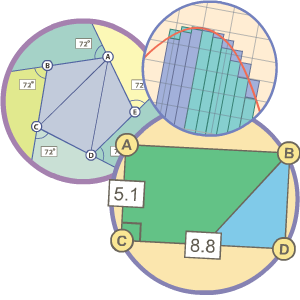The goal of the Common Core State Standards (CCSS) is to provide students with critical knowledge and skills for success in the 21st century. Gizmos help students of all ability levels to develop the deep knowledge that the Common Core's content standards demand, while enhancing their mathematical expertise, as outlined in the Standards for Mathematical Practice.

Gizmos allow students to go beyond memorizing facts, formulas and mnemonics, and instead enable students to develop a deep conceptual understanding of underlying principles.

Explore how Gizmos help teachers and students meet the challenge of the Common Core.

The Standards for Mathematical Content are a balanced combination of procedure and understanding.

The Common Core State Standards for Mathematics, About the Standards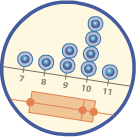### How do Gizmos Support the Standards for Mathematical Content?

The Common Core's content standards not only define what students should know in mathematics, but also explore how they learn it. Gizmos are highly interactive, online simulations that help students develop a deep understanding of challenging mathematical topics and the "hows" and "whys" behind them. With Gizmos, students don't just read or listen or watch. Instead, they are actively engaged, manipulating key variables and working with multiple visual representations to truly make sense of the math they are learning.

See how Gizmo simulations and Lesson Materials help students develop deep mathematical understanding. Pictured Gizmo: Distance-Time Graphs.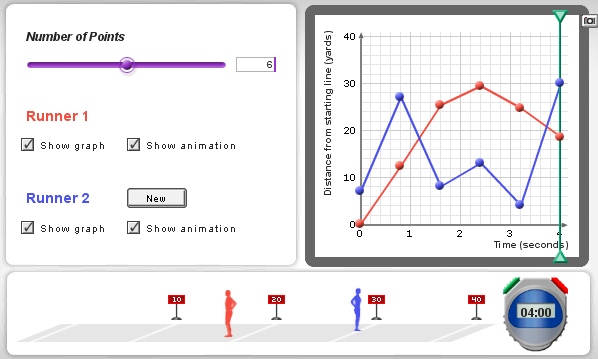The Gizmo simulation provides the foundation for student learning at ExploreLearning.com. In the Distance-Time Graphs Gizmo, students can create a graph of a runner's position versus time, and watch the runner complete a 40-yard dash based on the graph they made. They can add a second runner, as shown above, and connect real-world meaning to the intersection of the two graphs.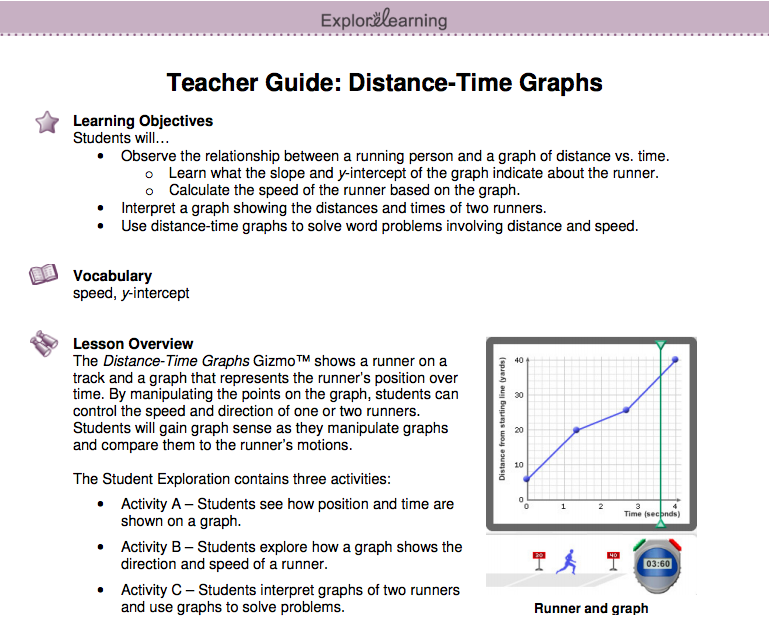The Teacher Guide provides educators with a roadmap for teaching with each Gizmo, including mathematical background information for additional content knowledge, learning objectives for students, lesson overview and suggested lesson sequence.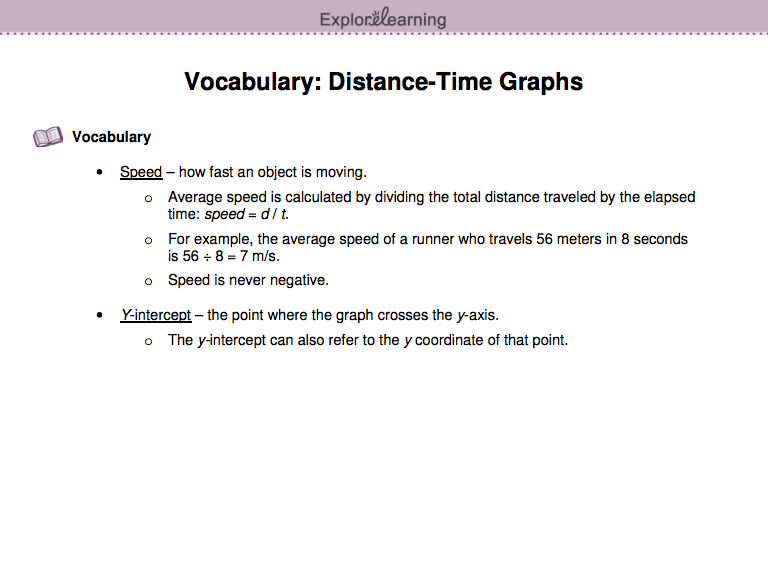The Vocabulary Sheet introduces key terminology that students will encounter working with the Gizmo concepts.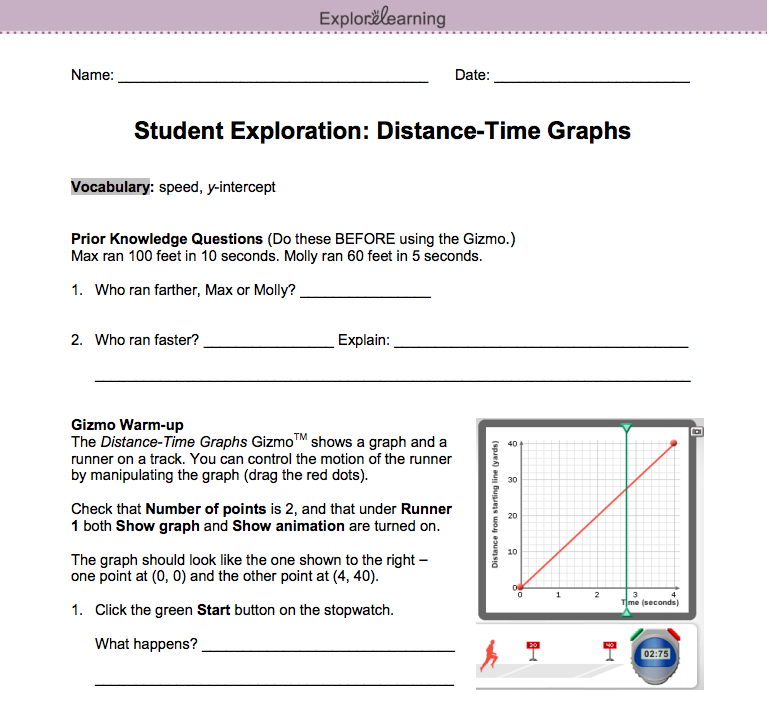The Student Exploration Sheet is an inquiry-based set of activities to help students progressively develop a deeper understanding of concepts.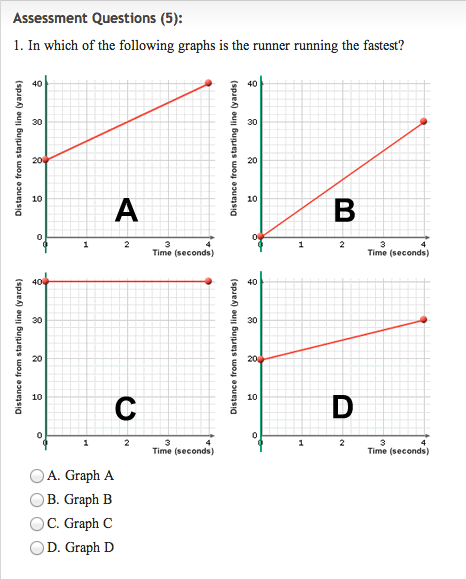The five Assessment Questions provide a check of student understanding with immediate feedback for students and real-time reporting for teachers.

The Standards for Mathematical Practice describe varieties of expertise that mathematics educators at all levels should seek to develop in their students.

The Common Core State Standards for Mathematics, About the Standards

## How do Gizmos Support the Standards for Mathematical Practice?

The Standards for Mathematical Practice (SMP) rest on important "processes and proficiencies" with longstanding support from leading mathematics education organizations and researchers. Gizmos are ideal for incorporating these standards into the learning experience. With accompanying Lesson Materials providing the guiding questions, students use Gizmos to develop both the knowledge and the general mathematical capabilities that lead to long-term success.

##### Standards for Mathematical Practice (SMP):
1. Make sense of problems and persevere in solving them.
2. Reason abstractly and quantitatively.
3. Construct viable arguments and critique the reasoning of others.
4. Model with mathematics.
1. Use appropriately tools strategically.
2. Attend to precision.
3. Look for and make use of structure.
4. Look for and express regularity in repeated reasoning.

Every mathematics Gizmo in our library supports teaching the Standards for Mathematical Practice. Below are some samples from a variety of grade levels and topics.

## In-Depth Exploration of the Practices: Function Machines Gizmo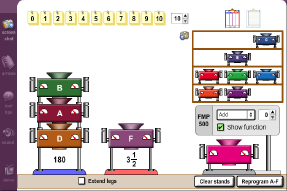Consider the Function Machines Gizmo. In this Gizmo, students are introduced to the concept of functions and function tables, using colorful input-output machines. Students program machines with function rules to create input-output tables. In addition, students have the ability to stack machines, create composite functions, and even program their own machines!

In addition to supporting the Common Core Content Standards 5.OA.3, 8.F.1, F.IF.1, and F.BF.4.a., the Function Machines Gizmo supports almost all of the Standards of Mathematical Practice:

Standards for Mathematical Practice How the Function Machines Gizmo Supports the Standard
1 Make sense of problems and persevere in solving them. Students are challenged to determine what a machine does, or 'solve' it, based on input-output pairs. Challenging problems can be created by stacking machines.
2 Reason abstractly and quantitatively. Students use abstract reasoning while looking at number patterns on charts and graphs. Students use quantitative reasoning to determine each machine's the specific function rule.
3 Construct viable arguments and critique the reasoning of others. Students discuss methods of solving machines and interpreting input-output data. Students can challenge each other to solve machines they have programmed.
4 Model with mathematics. Many real-world situations can be modeled with the Function Machines Gizmo, such as how revenue and profit depend on sales, or how distance traveled depends on time.
5 Use appropriate tools strategically. Several tools are available to help students solve their function machines. Students can program a machine to see if its output matches a mystery machine. They can also view machine input-output pairs on a table or plotted on a graph.
6 Look for and make use of structure. Patterns of input-output values help students determine how each machine functions.
8 Look for and express regularity in repeated reasoning. Students identify the pattern of the function machines to come up with rules, and develop strategies for solving new machines.

PARCC tests will be engaging and will test the critical-thinking and problem-solving skills students need to succeed in school and life.

New Vision of Assessment, PARCC

Smarter Balanced assessments will include extended response and technology-enhanced items, as well as performance tasks that allow students to demonstrate critical-thinking and problem-solving skills.

Sample Assessment Items, Smarter Balanced

### How do Gizmos Support the Common Core Assessments?

The Partnership for the Assessment of Readiness for College and Careers (PARCC) and the Smarter Balanced Assessment Consortium (SBAC) are developing online assessments to measure students' understanding of the standards.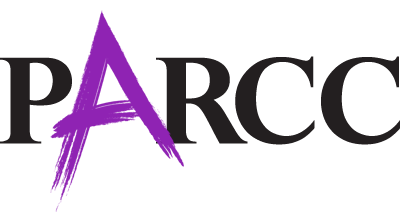Gizmos help prepare students for success on these assessments by providing experiences with the rigor and depth of understanding required. With Gizmos, students engage in complex tasks that require problem solving, critical thinking, modeling, data analysis, and information literacy. Students reason from the evidence and draw logical conclusions.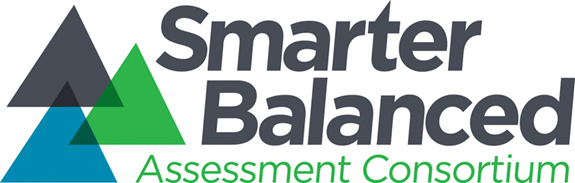In addition, many sample test items feature interactive tools - think 'mini-Gizmos' - directly integrated into the questions. These 'Gizmo-like' questions feature similar content and functions as the simulations in our library. Gizmos provide excellent preparation for these types of technology-enhanced questions.

Using the Fraction Artist 1 Gizmo, students learn that a fraction represents a relationship between a part and the whole, compare fractions and develop an understanding of equivalent fractions.

PARCC

Sample Test Item: This sample assessment item asks students to illustrate the fraction of a farmer's field that is planted by dragging a bean into each planted section.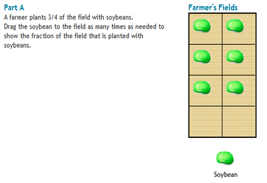Gizmo: This Gizmo allows students to divide a rectangular canvas in various ways and then 'paint' parts of it. The painting below is 6/8 (or 3/4) yellow.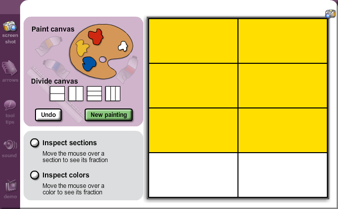SBAC

Sample Test Item: This sample assessment item asks students to divide a rectangle into equally sized pieces and then shade a specified fraction of them.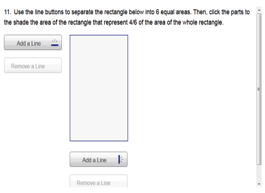Gizmo: This Gizmo allows students to divide a rectangular canvas in various ways and then 'paint' parts of it. The painting below is 4/6 (or 2/3) blue.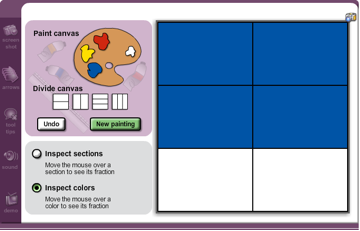With the Distance-Time Graphs Gizmo, students can observe the relationship between a runner's motion and a graph of distance versus time. They learn what the slope indicates and can calculate the speed of the runner based on the graph.

PARCC

Sample Test Item: This sample test item asks students to compare the speed of four objects based on distance/time data in graphs or tables.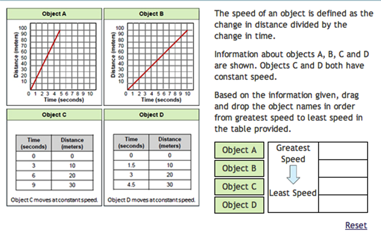Gizmo: The Gizmo shown below has one runner completing a 40-yard dash at a constant speed. The activity asks students to record the speed and time in a table.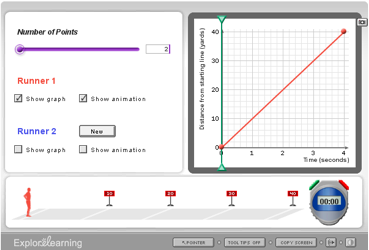SBAC

Sample Test Item: This sample test item shows runners in a 400-meter hurdles race. Students are asked to describe what is happening in this race.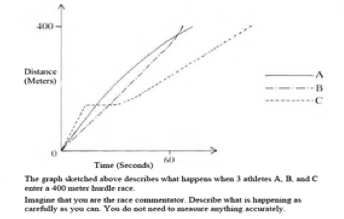Gizmo: This Gizmo scenario has two runners that vary their speeds throughout the race. The activity asks students to describe each runner's motion at each interval.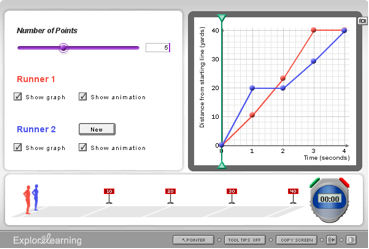The Quadratics in Vertex Form Gizmo provides good practice for the Common-Core-related assessments. Students can practice with a dynamic graph to see how changes to the function's parameters affect the shape, orientation, and location of the graph.

PARCC

Sample Test Item: This sample test item asks students to use sliders to create the graph of a specified quadratic function.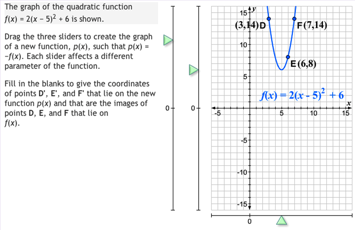Gizmo: In the Quadratics in Vertex Form Gizmo, students use sliders to graph quadratic functions and to explore the effects of varying parameters in the equation.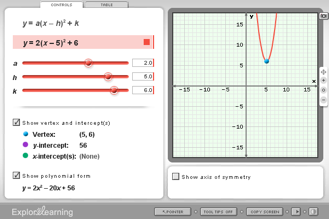SBAC

Sample Test Item: This sample assessment item asks students to shift the parabola's location to match a given quadratic function.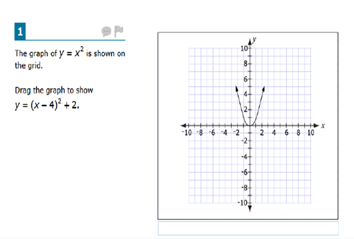Gizmo: The Quadratics in Vertex Form Gizmo also allows students to drag the parabola on the graph directly, and investigate how this affects the equation.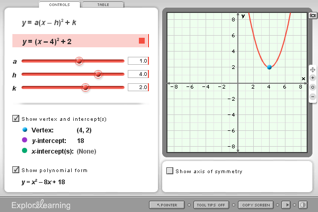* Copyright 2010 National Governors Association Center for Best Practices and Council of Chief State School Officers. All rights reserved.